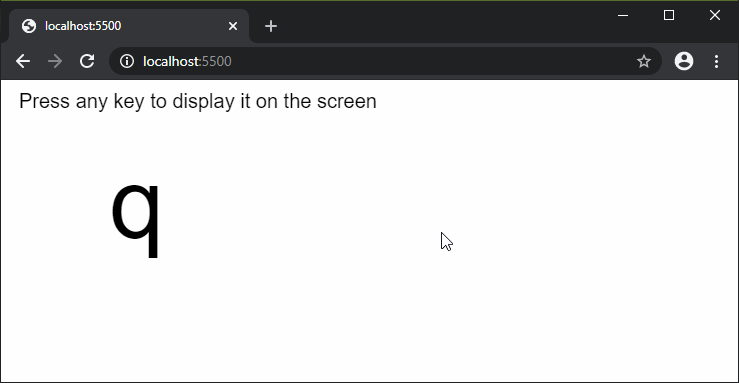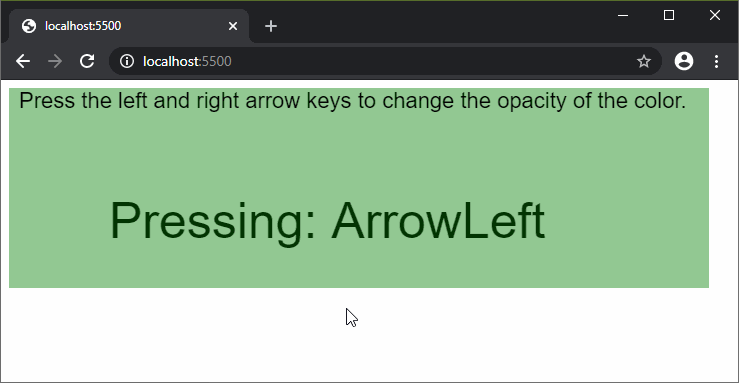Open In App
Related Articles
• Write an Interview Experience
• p5.js
• p5.js Introduction

# p5.js keyPressed() Function

The keyPressed() function is invoked whenever a key is pressed. The most recently typed ASCII key is stored into the ‘key’ variable, however it does not distinguish between uppercase and lowercase characters. The non-ASCII character codes can be accessed in the ‘keyCode’ variable with their respective names.
Holding down a key may cause multiple keyPressed() calls. This is due to how the Operating System handles keypressed and depends on how the computer is configured. A browser may have its own default behavior attached to various keys. This can be prevented by adding “return false” to the end of the method.
Syntax:

`keyPressed()`

Parameters: This method does not accept any parameters.
Below examples illustrate the keyPressed() function in p5.js:
Example 1:

## javascript

 `function` `setup() {``  ``createCanvas(600, 200);``  ``textSize(20);``  ``text(``"Press any key to display it "``          ``+ ``"on the screen"``, 10, 20);``}`` ` `function` `keyPressed() {``  ``clear();``  ``textSize(20);``  ``text(``"Press any key to display it "``          ``+ ``"on the screen"``, 10, 20);``  ``textSize(100);``  ``text(key, 100, 150);``}`

Output:Example 2:

## javascript

 `let opac = 128;`` ` `function` `setup() {``  ``createCanvas(700, 200);``  ``background(0, 128, 0, opac);``  ``textSize(22);``  ``text(``"Press the left and right arrow"``        ``+ ``" keys to change the opacity"``        ``+ ``" of the color."``, 10, 20);``}`` ` `function` `keyPressed() {``  ``clear();`` ` `  ``textSize(50);``  ``text(``"Pressing: "` `+ key, 100, 150);`` ` `  ``// Reduce opacity if the left arrow is pressed``  ``if` `(key == ``"ArrowLeft"` `&& opac > 0)``    ``opac -= 20;``  ``// Increase opacity if the left arrow is pressed``  ``else` `if` `(key == ``"ArrowRight"` `&& opac < 255)``    ``opac += 20;`` ` `  ``// Set the new background color``  ``background(0, 128, 0, opac);`` ` `  ``textSize(22);``  ``text(``"Press the left and right arrow"``        ``+ ``" keys to change the opacity"``        ``+ ``" of the color."``, 10, 20);``}`

Output:Online editor: https://editor.p5js.org/
Environment Setup: https://www.geeksforgeeks.org/p5-js-soundfile-object-installation-and-methods/
Reference: https://p5js.org/reference/#/p5/keyPressed